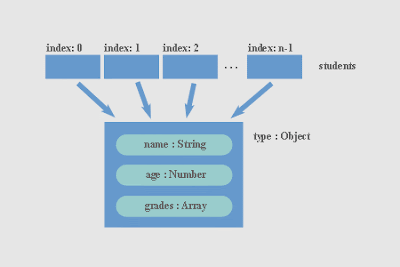In ActionScript 3 Arrays play very important role. This is powerfull class for storing and manipulating different type of values. If I have only one type of data, for example, only Strings, I will use Vector class which is almost exaclty like Array class, but it can store one data type only. Simple Array with different data types would be created like this:

``var student = new Array();student = "John";student = 22;student = "Elle";student = 21;``

Here I have strings representing names of students and numbers representing their age. I can trace all info at once with a loop:

``for (var i:uint = 0; i<student.length; i++) {    trace("info : "+student[i]);}``

Since my collection of data have clear "units" where single unit holds data for single student I need to structure data differently so I can take advantage of language for easier data manipulation. In this particular case indexed array is fine, but in some other cases it will be better and more efficient to use associative arrays or not use arrays at all.

For this example I'm using Objects to store data about students, one Object per student. Simplified example would look something like this:

``var st1 = new Object();st1.name = "John";st1.age = 22;st1.grades = [5, 4, 4, 3, 5, 3];``

Now I have my data structured like image shows.When data is structured like this it's easy to trace info and manipulate data. This example finds average grade for every student in students Array and outputs info for every student.

``var students = new Array();var st1 = new Object();st1.name = "John";st1.age = 22;st1.grades = [5, 4, 4, 3, 5, 3];students.push(st1);var st2 = new Object();st2.name = "Ella";st2.age = 20;st2.grades = [4, 5, 5, 4, 5];students.push(st2);for(var i:uint = 0; i < students.length; i++) {    trace((i+1)+".");    trace("NAME : "+students[i]["name"]);    trace("AGE : "+students[i]["age"]);    trace("grades : "+students[i]["grades"]);    var temp:Number = getAverage(students[i]);    trace("average grade :: "+temp);    trace("");}function getAverage(o:Object):Number{    var sum:Number = 0;    for (var j:uint = 0; j < o.grades.length; j++) {        sum = sum + o.grades[j];    }    return sum / o.grades.length;}``

You can use Array class methods to add new students // students.push(st3) // or remove them from array with splice. To remove John use // students.splice(0,1);

*_*